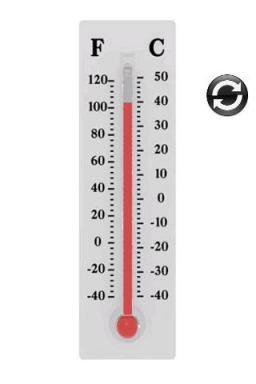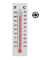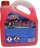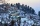# Laboratory

Mang Elio went to his laboratory at 7:00am
And recorded that the temperature was 11.07°C. At lunchtime, the temperature rose by 27.9°C but dropped by 8.05°C at 5:00 PM. What was the temperature at 5:00 PM?

t =  30.92 °C

### Step-by-step explanation:

$t=11.07+27.9-8.05=30.92\text{°C}$Did you find an error or inaccuracy? Feel free to write us. Thank you!## Related math problems and questions:

• WeatherWeather. At 5:00 am, the temperature was 28°C, and at noontime, it rose by 9°C. By 4:00 pm, it dropped 3°C, and by nighttime, it was 4°C less. What is the room temperature this time?
• OpheliaOphelia recorded the temperature of a cold store every two hours. 1. At 6 am, it was -4°C, and at 8 am, it was -1°C. By how much did the temperature rise? 2. The temperature went up by 5°C in the next two hours. .What was the temperature at 10 am?
• Temperature variationsToday's temperature was 80 degrees, and then the temperature dropped 10 degrees. Then it dropped 15 degrees again, then the next day, the temperature went up 2 degrees. What would the temperature be?
• The temperature 11The temperature on Sunday was 4°C. The temperature dropped 8°C on Monday and twice as much on Tuesday. What is the temperature on Tuesday?
• Celsius 25 degrees Celsius at midday dropped 12°Celsius degree by evening. What is the temperature?
• At midmorningThe temperature at midmorning was 31°C. Early in the evening, the temperature dropped by 5°C. What was the resulting temperature?
• Now isNow is 5°C morning. What will the temperature be if i. It rises by 12°C ii. It falls by 15°C
• Change in temperatureStarting temperature is 21°C, the highest temperature is 32°C. What is the change in temperature?
• Temperature differenceLibya's highest temperature was recorded at 58 degrees Celsius, and the lowest was recorded at -88 degrees Celsius. What is the temperature difference?
• Temperature up and dowmAt 6 AM, the temperature is 19.4°C. Between 6 AM and noon, the temperature rises 3.8°C. Between noon and 6 PM, the temperature falls 2.5°C. What is the temperature at 6 PM?
• The temperature 10The temperature in a freezer is -15°C, and it increases by 3°C.
• 8 degrees8 degrees Celsius it cools down by 9 degrees Celsius. What is the resultant temperature?
• The lowestThe lowest temperature on Thursday was 20°C. The lowest temperature on Saturday was 12°C. What was the difference between the lowest temperatures?
• Temperature rise and fallOn Friday, the temperature was 82°F. The temperature changed by –2°F on Saturday, and then it changed by 5°F on Sunday. What was the temperature on Sunday? How did the temperature change? Note we consider the mathematical problem formulated in this way to
• The temperatureThe temperature at 1:00 was 10 F. Between 1:00 and 2:00, the temperature dropped 15F. Between 2:00 and 3:00, the temperature rose 3F. What is the temperature at 3:00?
• Temperature 13Work out the temperature after each change. The temperature starts at 6°C and falls by 13°C. The temperature starts at 2°C and falls by 8°C
• Cooler placeThe temperature recorded in Simla is -4°C and in Kufri is -6°C on the same day which place is cooler on the day?